# The car is travelling at a speed of 100 km/h and as it decelerates at 2...

The car is travelling at a speed of 100 km/h and as it decelerates at 2 m/s^2. If the rain is falling at 6 m/s in the direction shown, determine the velocity and acceleration of the rain as seen by the driver.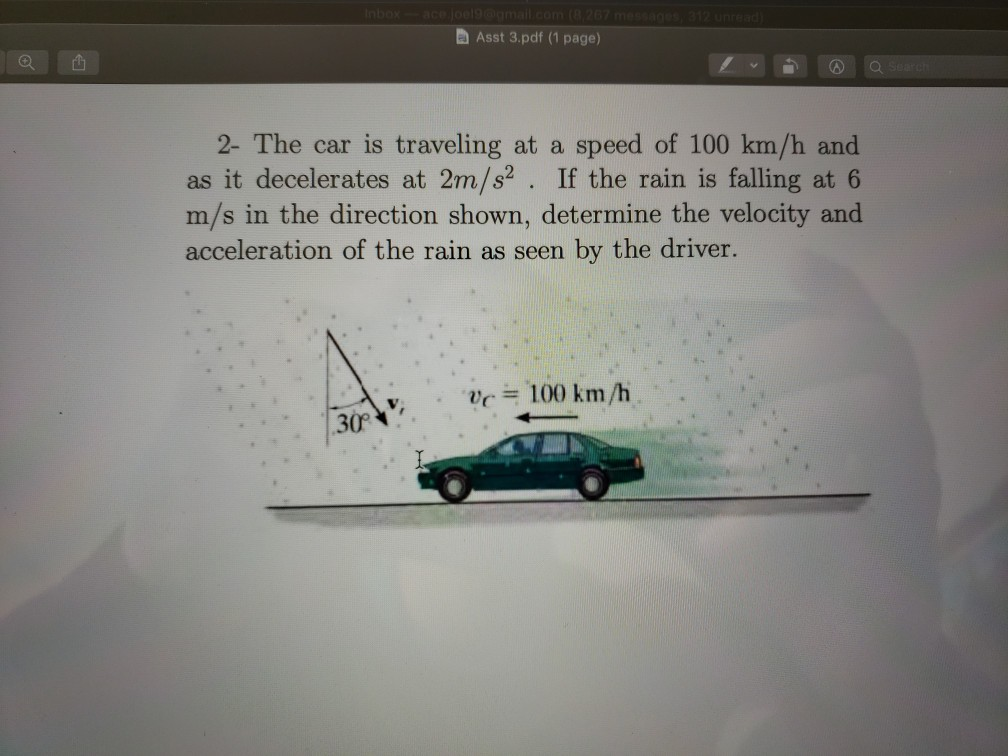Asst 3.pdf (1 page) 2- The car is traveling at a speed of 100 km/h and as it decelerates at 2m/s2. If the rain is falling at 6 m/s in the direction shown, determine the velocity and acceleration of the rain as seen by the driver. vc = 100 km/h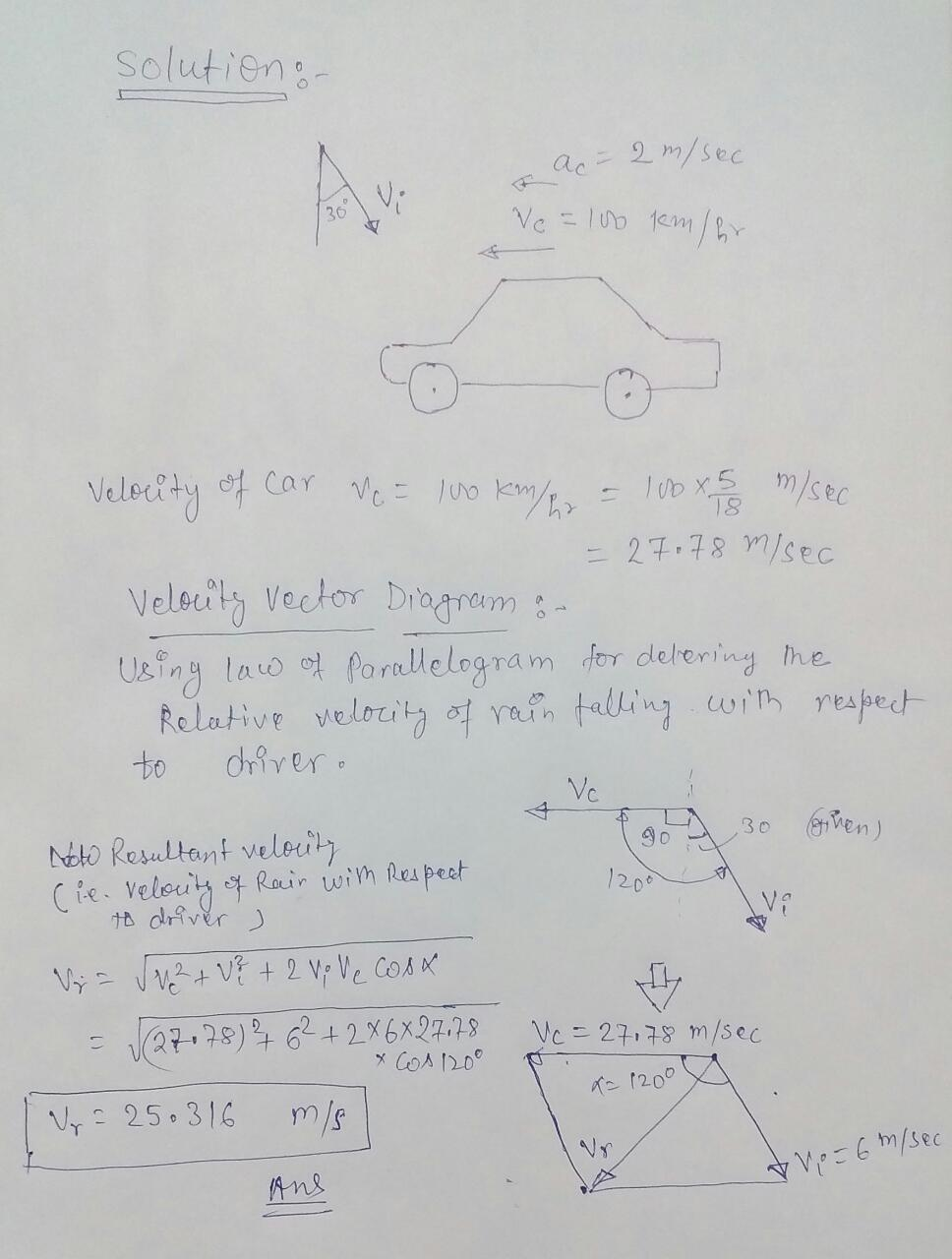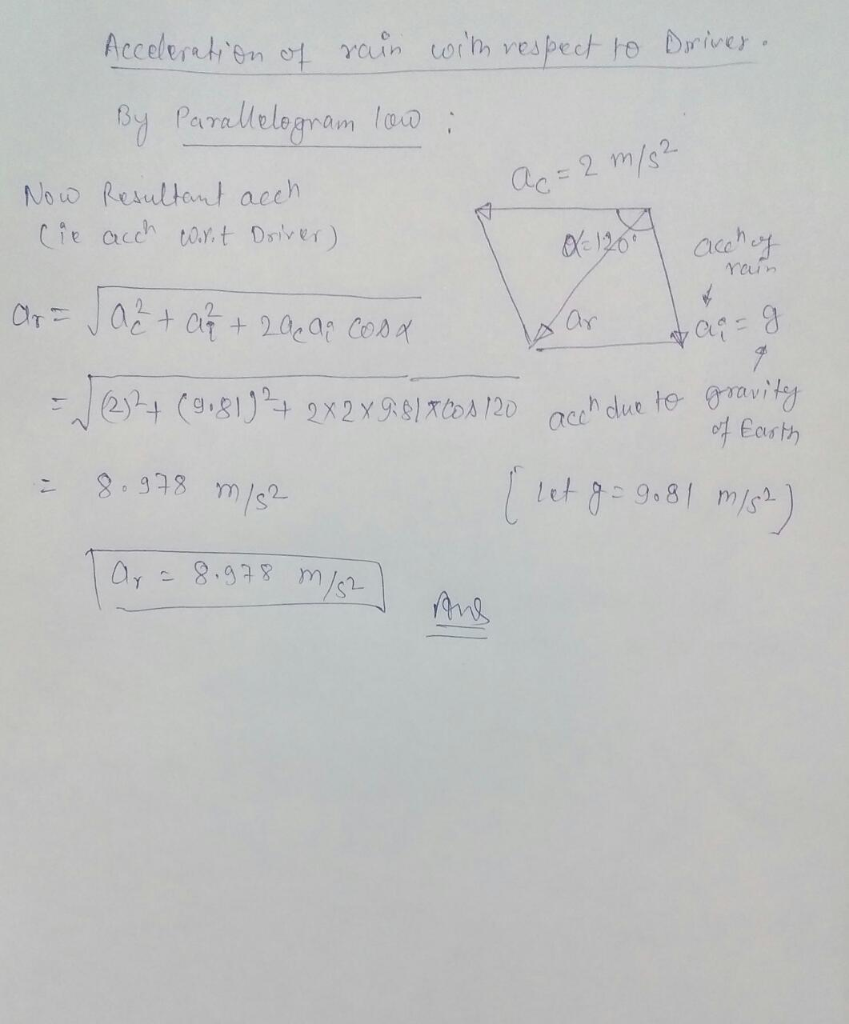#### Earn Coin

Coins can be redeemed for fabulous gifts.

Similar Homework Help Questions
• ### 11.78 A car is traveling at a constant speed of 54 km/h when its driver sees...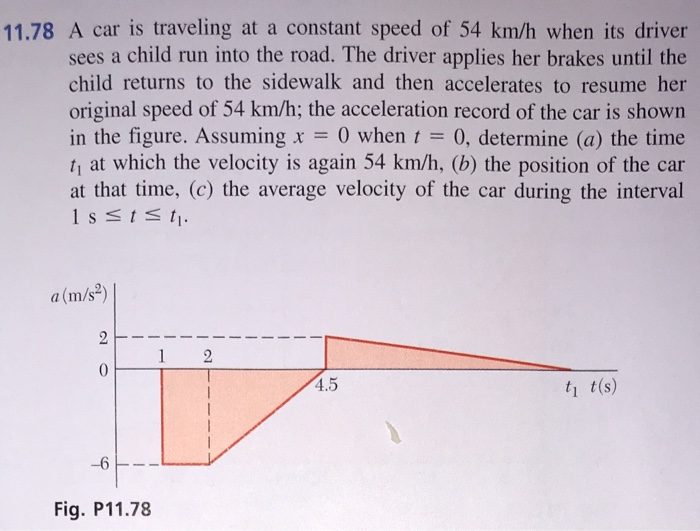11.78 A car is traveling at a constant speed of 54 km/h when its driver sees a child run into the road. The driver applies her brakes until the child returns to the sidewalk and then accelerates to resume her original speed of 54 km/h; the acceleration record of the car is shown in the figure. Assuming x = 0) when t = 0, determine (a) the time t, at which the velocity is again 54 km/h, (b) the position...

• ### The speed limit in a school zone is 38 km/h

The speed limit in a school zone is 38 km/h. A driver traveling at this speed sees a child run onto the road 16 m ahead of his car. He applies the brakes, and the car decelerates at a uniform rate of 8.5 m/s2. If the driver's reaction time is 0.55 s, What is the stopping distance of the vehicle?

• ### The speed limit in a school zone is 45 km/h. A driver traveling at this speed...

The speed limit in a school zone is 45 km/h. A driver traveling at this speed sees a child run onto the road 20 m ahead of his car. He applies the brakes, and the car decelerates at a uniform rate of 11.0 m/s2. If the driver's reaction time is 0.55 s, will the car stop before hitting the child?What is the stopping distance of the vehicle?

• ### a car is travelling on with speed v=72 km/h around a banked cure of diameter d=...

a car is travelling on with speed v=72 km/h around a banked cure of diameter d= 190 m calculate the following: a. the centripetal acceleration of the car. b.the banked angle to keep the car from sliding assume no friction. c.assume the curve is leveled horizontally , determine the coefficient of static friction to keep the car from sliding d.Calculate the centeripetal force at the given spedd on a car of mass, m=1000 kg and a truck of mass m=12500...

• ### A car travels due east with a horizontal speed of 51.8 km/h. Rain is falling vertically...

A car travels due east with a horizontal speed of 51.8 km/h. Rain is falling vertically with respect to Earth. The traces of the rain on the side windows of the car make an angle of 67.8◦ with the vertical. Find the magnitude of the velocity (km/h) of the falling rain before it hits the car. Hint: The three velocity vectors are (1) the velocity of the car with respect to the ground (horizontal), (2) the velocity of the rain...

• ### A car is moving on a city street at a speed of 30 km/h. The driver...

A car is moving on a city street at a speed of 30 km/h. The driver sees a red traffic signal ahead and slams on the brakes, providing an acceleration that has magnitude 6.0 m/s2. The driver's reaction time is 0.50 s. (a) Determine the displacement of the car until it stops. ___m (b) Repeat Part (a) if the initial speed is 60 km/h and the braking acceleration is the same as in Part (a). ___m

• ### A car travels due east with a speed of 42.0 km/h

A car travels due east with a speed of 42.0 km/h. Raindrops are falling at a constant speed vertically with respect to the Earth. The traces of the rain on the side windows of the car make an angle of 70.0° with the vertical. Find the velocity of the rain with respect to the following reference frames. (Enter the magnitude of the velocity.)(a) the car(b) the Earth

• ### A car travels due east with a speed of 35.0 km/h. Raindrops are falling at a...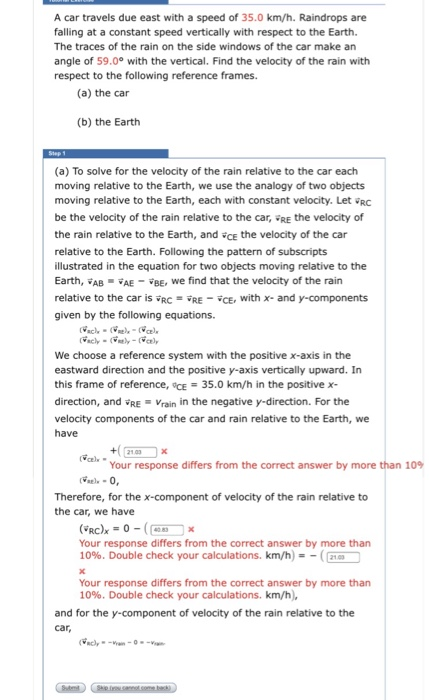A car travels due east with a speed of 35.0 km/h. Raindrops are falling at a constant speed vertically with respect to the Earth. The traces of the rain on the side windows of the car make an angle of 59.0° with the vertical. Find the velocity of the rain with respect to the following reference frames (a) the car (b) the Earth (a) To solve for the velocity of the rain relative to the car each moving relative to...

• ### The speed limit in a school zone is 40 km/h

The speed limit in a school zone is 40 km/h. A driver traveling at this speed sees a child run into the road 23 m ahead of hs car. He applies the brakes, and the car decelerates at a uniform rate of 8.0m/s^2. if the driver's reaction time is .25s, will the car stop before hitting the child?

• ### 1. A car accelerates from zero to 105 km/h in 5.21 s. What is its average...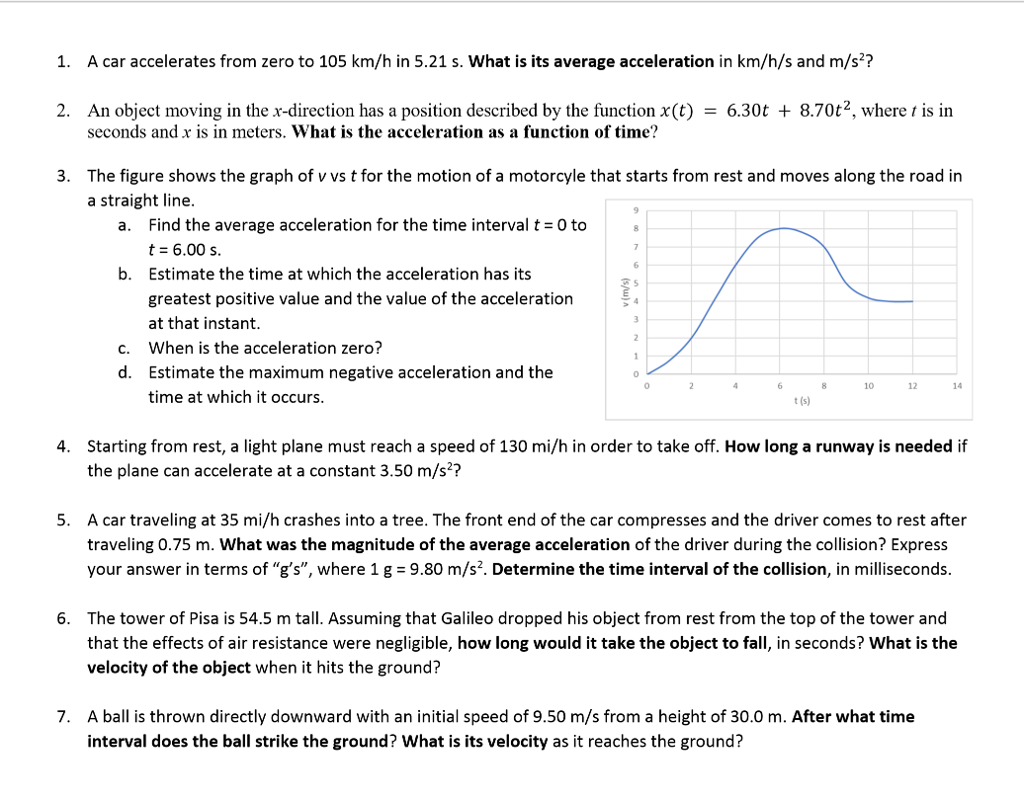1. A car accelerates from zero to 105 km/h in 5.21 s. What is its average acceleration in km/h/s and m/s? 2. An object moving in the x-direction has a position described by the function x(t) - 6.30t + 8.70t2, where t is in seconds and x is in meters. What is the acceleration as a function of time? The figure shows the graph of v vs t for the motion of a motorcyle that starts from rest and moves...

Free Homework App